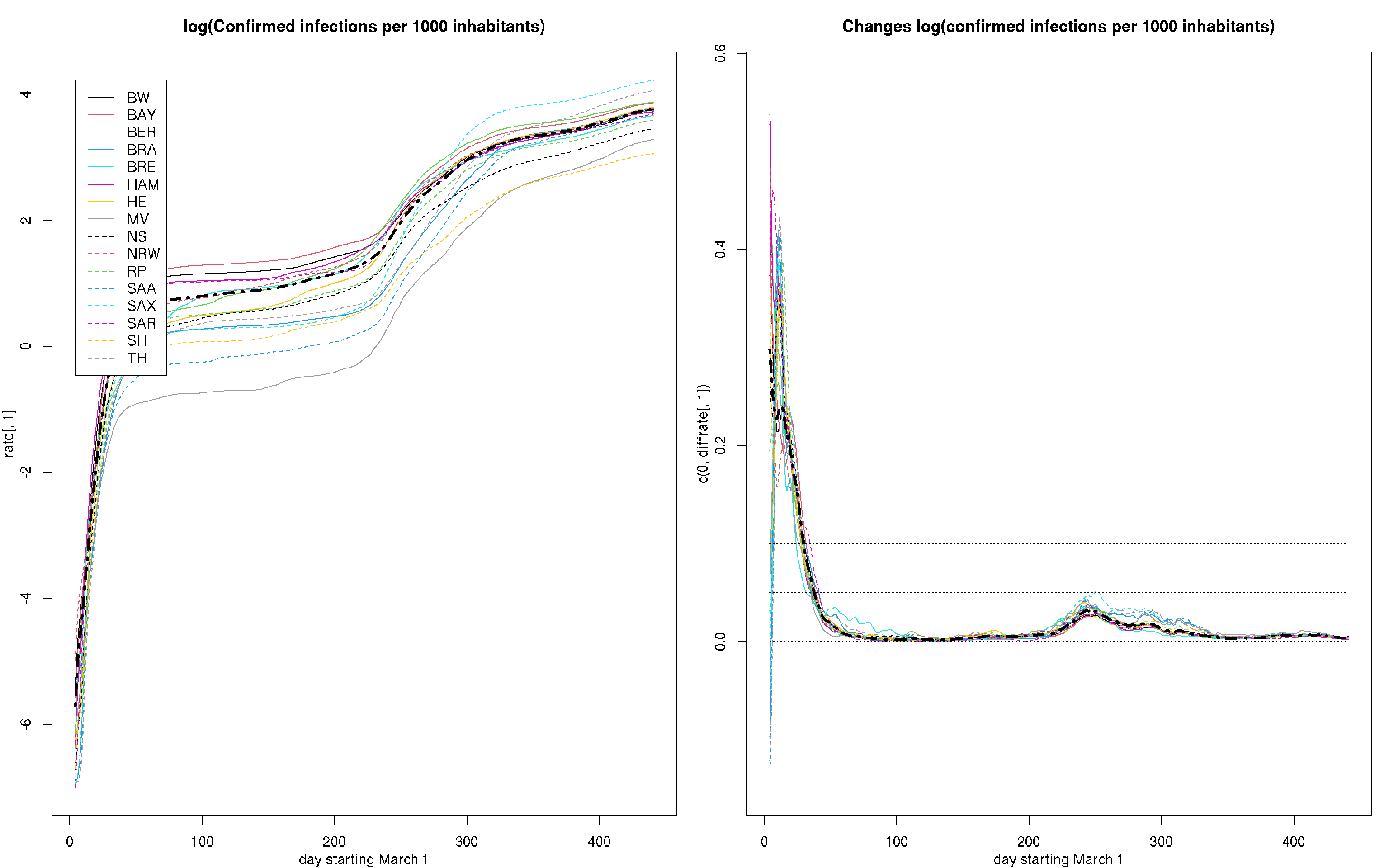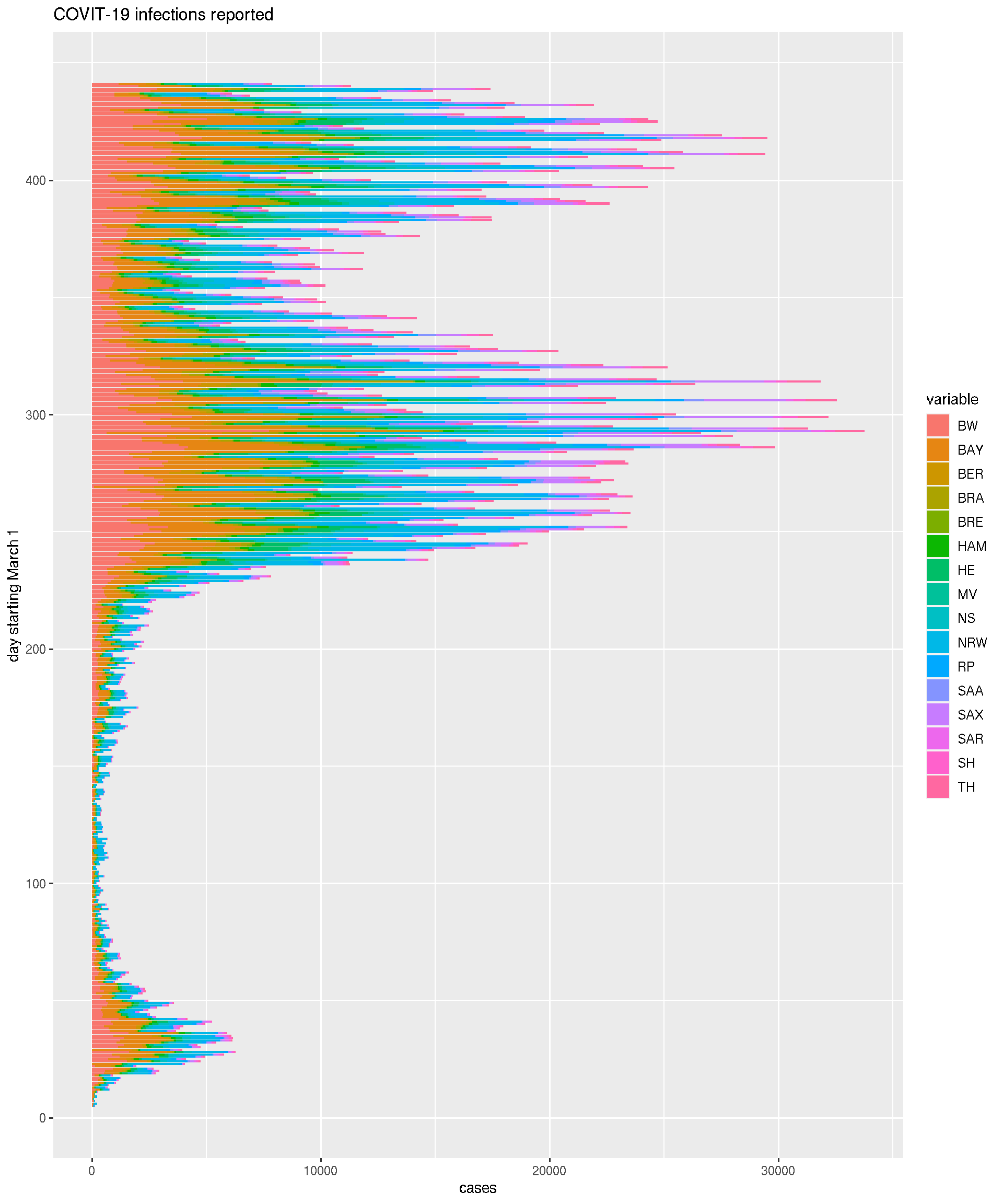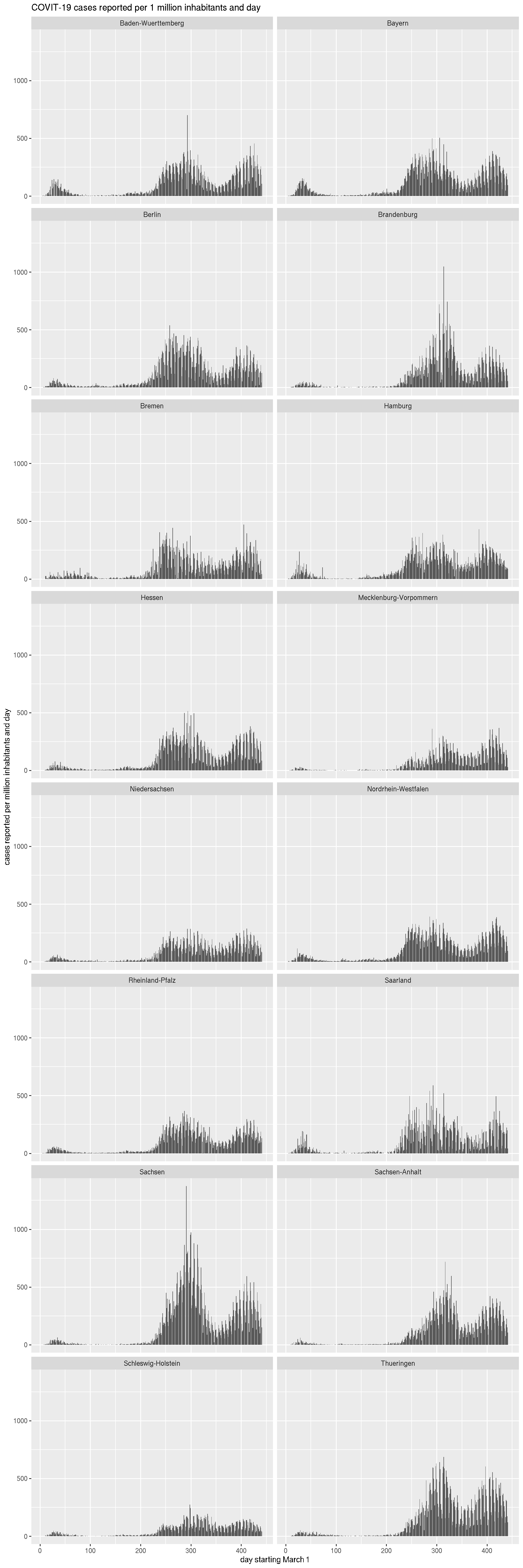## General remarks

This analysis is based on the daily updated numbers of confirmed infections provided by the Robert Koch Institute in their situation reports.

``````data <- read.table(datafile,header=TRUE)
pop <- unlist(data[1,-1])
poptotal <- sum(pop)
dates <- as.matrix(data[-1,1])
rd <- range(dates)
cases <- data[-1,-1]
casestotal <- apply(cases,1,sum)
state <- names(cases)
rate <- sweep(cases,2,pop,"/")
ratetotal <- casestotal/poptotal
rate[rate < 1e-3] <- 1e-3
diffrate <- apply(rate,2,diff)
diffcases <- apply(cases,2,diff)``````

Note that in the few instances of negative new cases / deaths reported the cummulative numbers from the preceeding day were used.

For modeling we use local polynomial regression as implemented in function locpoly in R package KernSmooth. We employ a Gaussian kernel with bandwidth (kernel standard deviation) 1.5 days for estimating rates and 2.5 for estimating derivatives. Polynomial orders are 1 and 2, respectiviley, to minimize boundary bias. dates are in days after March 1.

Please note that the estimated curves for small number of reported cases carry a high variability. This is especially due for early dates and states with low population (BRE, SAR). Problems in the data like underreporting and reporting bias around weekends are not modeled.

## Confirmed infections

The data reported by the RKI show a few irregularities in form of a negative difference in the number of cases / deaths in compared to the preceeding day. In such cases the numbers used here are adjusted to yield a difference of 0. The most extreme case I observed was on May 27, when the Ostalbkreis (BW) reported a difference of -410 cases.

``````bw <- 1.5
dbw <- 2.5
rlcurves <- dlcurves <- list(NULL)
rlylim <- dlylim <- NULL
for (i in 1:16) {
rlcurves[[i]] <- locpoly(dates,log(pmax(1e-4,rate[,i])),bandwidth=bw,degree=1)
rlylim <- range(rlylim,rlcurves[[i]]\$y)
dlcurves[[i]] <- locpoly(dates,log(pmax(1e-4,rate[,i])),bandwidth=dbw,drv=1,degree=2)
dlylim <- range(dlylim,dlcurves[[i]]\$y)
}
rltotcurve <- locpoly(dates,log(pmax(1e-4,ratetotal)),bandwidth=bw,degree=1)
dltotcurve <- locpoly(dates,log(pmax(1e-4,ratetotal)),bandwidth=dbw,drv=1,degree=1) ``````
``````par(mfrow=c(1,2),mar=c(3,3,3,1),mgp=c(2,1,0))
plot(dates,rate[,1],ylim = rlylim,type="n",xlab="day starting March 1")
for(i in 1:16)
lines(rlcurves[[i]]\$x,rlcurves[[i]]\$y,type="l",col=i,lty=1+(i-1)%/%8)
lines(rltotcurve\$x,rltotcurve\$y,lty=4,lwd=3)
legend(4,rlylim,state,col=1:16,lty=1+(0:15)%/%8)
title("log(Confirmed infections per 1000 inhabitants)")

plot(dates,c(0,diffrate[,1]),ylim = dlylim,type="n",xlab="day starting March 1")
for(i in 1:16) lines(dlcurves[[i]]\$x,dlcurves[[i]]\$y,type="l",col=i,lty=1+(i-1)%/%8)
lines(dltotcurve\$x,dltotcurve\$y,lty=4,lwd=3)
title("Changes log(confirmed infections per 1000 inhabitants)")

for(lvl in c(0,.05,.1)) lines(rd,c(lvl,lvl),lty=3)``````## Infections per day

``````library(ggplot2)
library(reshape2)
fdiffcases <- as.data.frame(diffcases)
fdiffcases\$dates <- dates[-1]
fdcases <- melt(fdiffcases, id.vars="dates")
f1 <- ggplot(fdcases, aes(dates,value))
f2 <- f1 + geom_bar(aes(fill=variable),position=position_stack(reverse=TRUE),stat="identity") +
labs(title="COVIT-19 infections reported", x="day starting March 1", y="cases") +
coord_flip() +  theme(legend.position = "right")
f2````````````fstate <- c("Baden-Wuerttemberg","Bayern","Berlin","Brandenburg",
"Bremen","Hamburg","Hessen","Mecklenburg-Vorpommern",
"Niedersachsen","Nordrhein-Westfalen","Rheinland-Pfalz","Sachsen-Anhalt",
"Sachsen","Saarland","Schleswig-Holstein","Thueringen")
fdpm <- as.data.frame(diffrate)*1000
fdpm\$dates <- dates[-1]
fd <- melt(fdpm, id.vars="dates")
fd\$states <- rep(fstate,rep(length(dates)-1,16))
f1 <- ggplot(fd, aes(dates,value))

f1 + geom_bar(stat="identity") + facet_wrap(~states,nrow=9) + labs(title="COVIT-19 cases reported per 1 million inhabitants and day", x="day starting March 1", y="cases reported per million inhabitants and day") ``````## Active cases

Active cases are here defined as cases that were reported within the last 14 days. This is slightly larger than the numbers reported by RKI (corresponding to approximately the last 11 days) and was chosen to minimize effects due to a weekly cycly of reporting biases observed in the data.

``````bw <- 1.50
dbw <- 2.5
inc <- 14 # 11 corresponds to RKI estimate of 23000 Recoveries on April 3
rlcurves <- dlcurves <- list(NULL)
rlylim <- dlylim  <- NULL
for (i in 1:16) {
rlcurves[[i]]<- locpoly(dates,log(pmax(1e-4,diff(c(rep(0,inc),rate[,i]),inc))),bandwidth=bw,degree=1)
rlylim <- range(rlylim,rlcurves[[i]]\$y)
dlcurves[[i]] <- locpoly(dates,log(pmax(1e-4,diff(c(rep(0,inc),rate[,i]),inc))),bandwidth=dbw,drv=1,degree=2)
dlylim <- range(dlylim,dlcurves[[i]]\$y)
}
rltotcurve <- locpoly(dates,log(pmax(1e-4,diff(c(rep(0,inc),ratetotal),inc))),bandwidth=bw,degree=1)
dltotcurve <- locpoly(dates,log(pmax(1e-4,diff(c(rep(0,inc),ratetotal),inc))),bandwidth=dbw,drv=1,degree=2) ``````

The left plot provides estimates of the logarithmic rate of of active cases while the right hand site displays its first derivative.

``````par(mfrow=c(1,2),mar=c(3,3,3,1),mgp=c(2,1,0))
plot(dates,rate[,1],ylim = pmin(rlylim,5),type="n",xlab="day starting March 1",ylab="")
for(i in 1:16)
lines(rlcurves[[i]]\$x,rlcurves[[i]]\$y,col=i,lty=1+(i-1)%/%8)
lines(rltotcurve\$x,rltotcurve\$y,lty=4,lwd=3)
legend(4,rlylim,c(state,"all"),col=c(1:16,1),lty=c(1+(0:15)%/%8,4),lwd=c(rep(1,16),3))
title("log(Active cases per 1000 inhabitants)")

plot(dates,c(0,diffrate[,1]),ylim = pmin(dlylim,0.2),type="n",xlab="day starting March 1",ylab="")
for(i in 1:16) lines(dlcurves[[i]]\$x,dlcurves[[i]]\$y,col=i,lty=1+(i-1)%/%8)
lines(dltotcurve\$x,dltotcurve\$y,lty=4,lwd=3)
#lines(dates[-1],diffrate[,i],type="l",col=i,lty=1+(i-1)%/%8)
#legend(5,dlylim,state,col=1:16,lty=1+(0:15)%/%8)
title("Changes log(Active cases per 1000 inhabitants)")
lines(rd,c(0,0),lty=3)
for(lvl in seq(-.2,.1,.05)[-5]) lines(rd,c(lvl,lvl),lty=3)``````